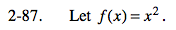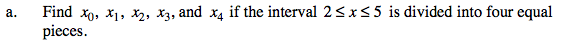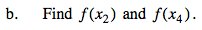### Home > PC > Chapter 2 > Lesson 2.3.2 > Problem2-87

2-87.
1. Let f(x) = x2. Homework Help ✎

1. Find x0, x1, x2, x3, and x4 if the interval 2 ≤ x ≤ 5 is divided into four equal pieces.

2. Find f(x2) and f(x4).$\text{Width between each piece}=\frac{\text{length of the interval}}{\text{Number of pieces}}$Use the information you found for x2 and x4 above to substitute into the given function.